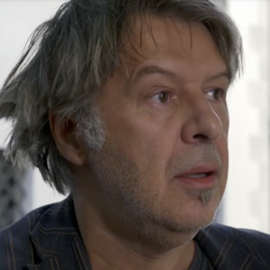# Constructions of balanced Boolean functions on even number of variables with maximum absolute value in autocorrelation spectra $<2^{{n/2}^{\star}}$

### Abstract

The autocorrelation properties of Boolean functions are closely related to the Shannon’s concept of diffusion and can be accompanied with other cryptographic criteria (such as high nonlinearity and algebraic degree) for ensuring an overall robustness to various cryptanalytic methods. In a series of recent articles [14,9,15], the design methods of $n$-variable balanced Boolean functions n is strictly even) with small absolute indicator $\Delta_f < 2^{n/2}$ have been considered. Whereas the two first articles managed to solve this problem for relatively large $n\geq 46$, a recent approach  has introduced a generic design framework achieving $\Delta_f < 2^{n/2}$ for even $n\geq 22$. Based on a suitable modification of the method of Rothaus, used to construct new bent functions from known ones, we provide a generic iterative framework for designing balanced functions satisfying the condition $\Delta_f < 2^{n/2}$ and having overall good cryptographic properties for any even n⩾12. Even though the problem of specifying functions having $\Delta_f < 2^{n/2}$ for smaller $n$ has been considered in [14,9,15] using various search algorithms, our method for the first time provides relatively simple iterative framework for variable spaces of more practical interest. Moreover, our approach can be efficiently applied to certain classes of initial functions (derived from partial spread bent functions) for deriving balanced functions with $\Delta_f < 2^{n/2}$ for relatively large $n$, namely for $n\geq 48$ satisfying $n \mod 4=0$ and $n\geq 54$ with $n\mod 4=2$. In the latter case, our nonlinearity bound is better than the one presented in .

Publication
Information Sciences